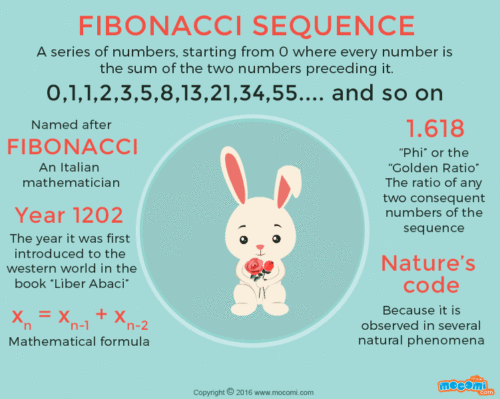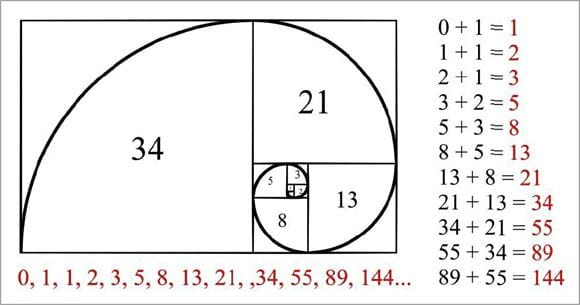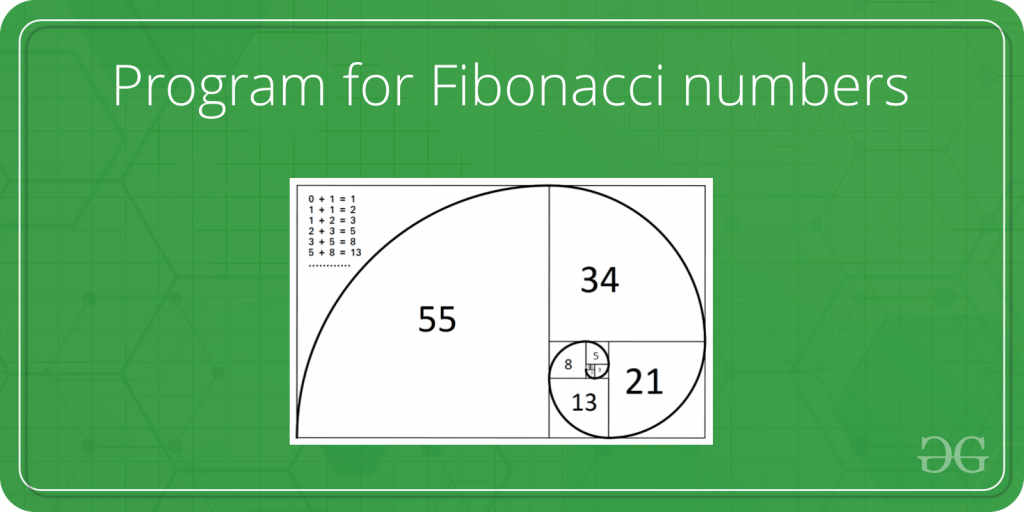## fibonacci_series

Fibonacci Sequence. The Fibonacci Sequence is the series of numbers: 0, 1, 1, 2, 3, 5, 8, 13, 21, 34, ... The next number is found by adding up the two numbers before it.

### 2 unstable releases

 0.2.0 Nov 3, 2019 Oct 31, 2019

#971 in Algorithms

430KB

# 2. Imgaes:

## below picture is the short story of Fibonacci Sequence# 3. Graphical Interpretation:# 5. Code Block:

``````

/* This program is for printing series of first 'N' (user given limit) Fibonacci Numbers on the console */

use std::io;

pub fn fibonacci() {

println!("\n    Please enter the quantity of Fibonacci number series\n    ");
let mut num = String::new();
let num : u32 = num.trim().parse().unwrap();

let mut first   : usize = 0;
let mut second  : usize = 1;
let mut initial : usize = 0;
let mut next    : usize;
println!("\n     The following is the Fibonacci series\n");
println!("    **************************************\n");
// for first N Fibonacci Series, we used 0..num Range pattern with num excluding
for _x in 0..num {
if initial <= 1 {
next = initial;
initial= 1+initial;
}

else{
next = first + second;
first = second;
second = next;
}
println!("      Number-{} : {},   \n",_x+1, next);
}
}

``````

~AlanPerils